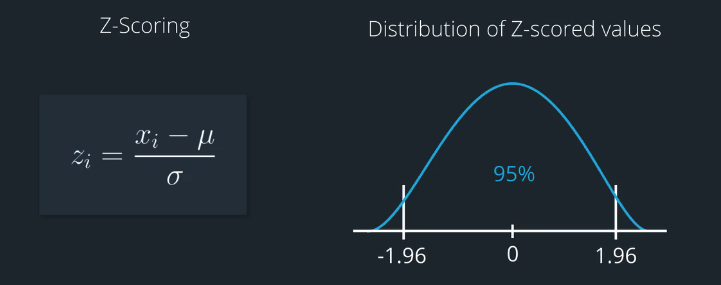# AI For Trading:Z Score (65)

## Z score

It's common for researchers to normalize alpha factors by subtracting the mean and dividing by the standard deviation. We can also refer to this process as Z-scoring because the result is a Z-score.

Z-scoring helps to standardize the data, so that it has a consistent range and distribution. This makes it easier to compare and combine more than one alpha factor.
Z-scoring有助于标准化数据，因此它具有一致的范围和分布。这样可以更轻松地比较和组合多个alpha因子。

For instance, if we assume a somewhat Gaussian distribution, then about 95 percent of the values in a Z-scored distribution would be roughly between negative two and positive two.Ranking

• Makes our alpha vectors more robust against outliers and noise
• Best to use when all alpha vectors are generated from the same stock universe

Z-Scoring

• Not robust aginst outliers and noise
• Useful to apply ranking and then z-scoring, when alpha vectors are generated from defferent stock universes.
• Standardized for all quants and portfolios.

## z-score quize

Check out the documentation for the zscore function in Zipline According to the documentation, what parameter can you set if you wish to exclude extreme values from the calculation of the mean and standard deviation?

B. groupby

## Install packages

import sys
!{sys.executable} -m pip install -r requirements.txt
import cvxpy as cvx
import numpy as np
import pandas as pd
import time
import os
import quiz_helper
import matplotlib.pyplot as plt
%matplotlib inline
plt.style.use('ggplot')
plt.rcParams['figure.figsize'] = (14, 8)

### data bundle

import os
import quiz_helper
from zipline.data import bundles
os.environ['ZIPLINE_ROOT'] = os.path.join(os.getcwd(), '..', '..','data','module_4_quizzes_eod')
ingest_func = bundles.csvdir.csvdir_equities(['daily'], quiz_helper.EOD_BUNDLE_NAME)
bundles.register(quiz_helper.EOD_BUNDLE_NAME, ingest_func)
print('Data Registered')

### Build pipeline engine

from zipline.pipeline import Pipeline
from zipline.pipeline.factors import AverageDollarVolume
from zipline.utils.calendars import get_calendar

universe = AverageDollarVolume(window_length=120).top(500)
engine = quiz_helper.build_pipeline_engine(bundle_data, trading_calendar)

### View Data

With the pipeline engine built, let's get the stocks at the end of the period in the universe we're using. We'll use these tickers to generate the returns data for the our risk model.

universe_end_date = pd.Timestamp('2016-01-05', tz='UTC')

universe_tickers = engine\
.run_pipeline(
Pipeline(screen=universe),
universe_end_date,
universe_end_date)\
.index.get_level_values(1)\
.values.tolist()

universe_tickers

## Get Returns data

from zipline.data.data_portal import DataPortal

data_portal = DataPortal(
bundle_data.asset_finder,
adjustment_reader=bundle_data.adjustment_reader)

## Get pricing data helper function

def get_pricing(data_portal, trading_calendar, assets, start_date, end_date, field='close'):
end_dt = pd.Timestamp(end_date.strftime('%Y-%m-%d'), tz='UTC', offset='C')
start_dt = pd.Timestamp(start_date.strftime('%Y-%m-%d'), tz='UTC', offset='C')

return data_portal.get_history_window(
assets=assets,
end_dt=end_dt,
bar_count=end_loc - start_loc,
frequency='1d',
field=field,
data_frequency='daily')

## get pricing data into a dataframe

returns_df = \
get_pricing(
data_portal,
universe_tickers,
universe_end_date - pd.DateOffset(years=5),
universe_end_date)\
.pct_change()[1:].fillna(0) #convert prices into returns

returns_df

## Sector data helper function

We'll create an object for you, which defines a sector for each stock. The sectors are represented by integers. We inherit from the Classifier class. Documentation for Classifier, and the source code for Classifier

from zipline.pipeline.classifiers import Classifier
from zipline.utils.numpy_utils import int64_dtype
class Sector(Classifier):
dtype = int64_dtype
window_length = 0
inputs = ()
missing_value = -1

def __init__(self):

def _compute(self, arrays, dates, assets, mask):
return np.where(
self.data[assets],
self.missing_value,
)
sector = Sector()

## We'll use 2 years of data to calculate the factor

Note: Going back 2 years falls on a day when the market is closed. Pipeline package doesn't handle start or end dates that don't fall on days when the market is open. To fix this, we went back 2 extra days to fall on the next day when the market is open.

factor_start_date = universe_end_date - pd.DateOffset(years=2, days=2)
factor_start_date

## Quiz 1

Create a factor of one year returns, demeaned, and ranked, and then converted to a zscore

from zipline.pipeline.factors import Returns
#TODO
# create a pipeline called p
p = Pipeline(screen=universe)
# create a factor of one year returns, deman by sector, then rank
factor = (
demean(groupby=Sector()). #we use the custom Sector class that we reviewed earlier
rank().
zscore()
)
# add the factor to the pipeline
p.add(factor, 'Momentum_1YR_demean_by_sector_ranked_zscore')

## visualize the pipeline

p.show_graph(format='png')

## run pipeline and view the factor data

df = engine.run_pipeline(p, factor_start_date, universe_end_date)
df.head()

## Quiz 2

What do you notice about the factor values?